JEE  >  Irodov Solutions: Optics of Moving Sources- 1

# Irodov Solutions: Optics of Moving Sources- 1 - I. E. Irodov Solutions for Physics Class 11 & Class 12 - JEE

Q.224. In the Fizeau experiment on measurement of the velocity of light the distance between the gear wheel and the mirror is l = 7.0 km, the number of teeth is z = 720. Two successive disappearances of light are observed at the following rotation velocities of the wheel: n = 283 rps and n2  = 313 rps. Find the velocity of light.

Ans. In the Fizean experiment, light disappears when the wheel rotates to bring a tooth in the position formerly occupied by a gap in the time taken by light to go from the wheel to the mirror and back. Thusdistance travelled = 2l Suppose the mth tooth after the gap has come in place of the latter. Then time taken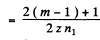sec. in the first case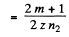sec in the second case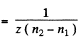Then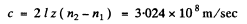Q.225. A source of light moves with velocity v relative to a receiver. Demonstrate that for v ≪ c the fractional variation of frequency of light is defined by Eq. (5.6a).

Ans. W hen v « c time dilation effect of relativity can be neglected (i.e. t’ ≈ t ) and we can use time in the reference frame fixed to the observer. Suppose the source emits short pulses with intervals T0 . Then in the reference frame fixed to the receiver the distance between two seccessive pulses is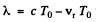when measured along the observation line.
Here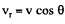is the projection of the source velocity on the observation line. The frequency of the pulses received by the observer is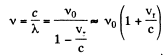(The formula is accurate to first order only)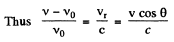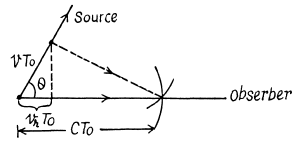The frequency increases when the source is moving towards the observer.

Q.226. One of the spectral lines emitted by excited He+ ions has a wavelength λ = 410 nm. Find the Doppler shift Δλ of that line when observed at an angle θ = 30° to the beam of moving ions possessing kinetic energy T = 10 MeV

Ans.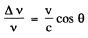from the previous problem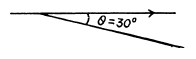But v λ = c gives an differentiation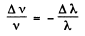So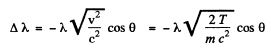on using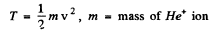We use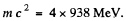Putting other values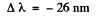Q.227. When a spectral line of wavelength λ = 0.59 [tm is observed in the directions to the opposite edges of the solar disc along its equator, there is a difference in wavelengths equal to δλ = 8.0 pm. Find the period of the Sun's revolution about its own axis.

Ans. One end of the solar disc is moving towards us while the other end is moving away from us. The angle θ between the direction in which the edges of the disc are moving and the line of observation is small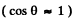. Thus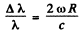where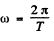is the angular velocity of the Sun. Thus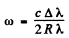So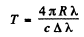Putting the values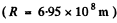we get         T = 24.85days

Q.228. The Doppler effect has made it possible to discover the double stars which are so distant that their resolution by means of a telescope is impossible. The spectral lines of such stars periodically become doublets indicating that the radiation does come from two stars revolving about their centre of mass. Assuming the masses of the two stars to be equal, find the distance between them and their masses if the maximum splitting of the spectral lines is equal to (Δλ/λ)m  = 1.2.10-4  and occurs every ζ = 30 days.

Ans. Maximum splitting of die spectral lines will occur when both of the stars are moving in the direction of line of observation as shown. We then have the equations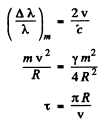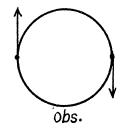From these we get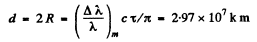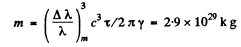Q.229. A plane electromagnetic wave of frequency coo  falls normally on the surface of a mirror approaching with a relativistic velocity V. Making use of the Doppler formula, find the frequency of the reflected wave. Simplify the obtained expression for the case V << c.

Ans. We define the frame S (the lab frame) by the condition of the problem. In this frame the mirror is moving with v elocity v (along say x - ax is) towards left and light of frequency ω0 is approaching it from the left. We introduce the frame S ' whose axes are parallel to those of S but which is moving with velocity v along x axis towards left (so that the mirror is at re st in S'). In S' the frequency of the incident ligh t is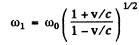In S.' the reflected light still has frequency ω0 but it is now moving towards left. When we transform back to S this reflected light has the frequency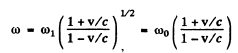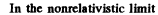Hence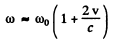Q.230. A radar operates at a wavelength λ = 50.0 cm. Find the velocity of an approaching aircraft if the beat frequency between the transmitted signal and the signal reflected from the aircraft is equal to Δv = 1.00 kHz at the radar location.

Ans.  From the previous problem, the beat frequency is clearly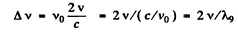Hence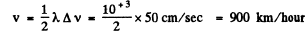Q.231. Taking into account that the wave phase ωt — kx is an invariant, i.e. it retains its value on transition from one inertial frame to another, determine how the frequency ω and the wave number k entering the expression for the wave phase are transformed. Examine the unidimensional case.

Ans. From the invariance of phase under Lorentz transformations we get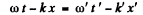Here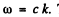The primed coordinates refer to the frame 5 ' which is moving to the right with velocity v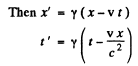Where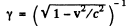Substituting and equating the coffiecients of t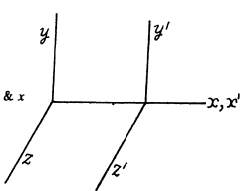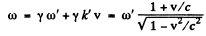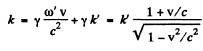Q.232. How fast does a certain nebula recede if the hydrogen line λ = 434 nm in its spectrum is displaced by 130 nm toward longer wavelengths?

Ans. Frome the previous problem using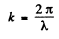We get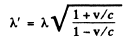Thus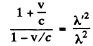or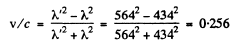Q.233. How fast should a car move for the driver to perceive a red traffic light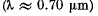as a green one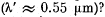Ans. As in the previous problem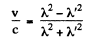so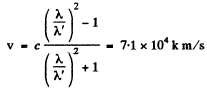Q.234. An observer moves with velocity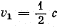along a straight line. In front of him a source of monochromatic light moves with velocity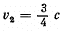in the same direction and along the same straight line. The proper frequency of light is equal to ωo. Find the frequency of light registered by the observer.

Ans. We go to the frame in which the observer is at rest. In this frame the velocity of the source of light is, by relativistic velocity addition foumula,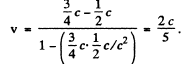When this source emits light of proper frequency ωo the frequency recorded by observer will be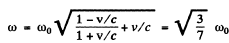Note that ω < ωas the source is moving away from the observer (red shift).

The document Irodov Solutions: Optics of Moving Sources- 1 | I. E. Irodov Solutions for Physics Class 11 & Class 12 - JEE is a part of the JEE Course I. E. Irodov Solutions for Physics Class 11 & Class 12.
All you need of JEE at this link: JEE

## FAQs on Irodov Solutions: Optics of Moving Sources- 1 - I. E. Irodov Solutions for Physics Class 11 & Class 12 - JEE

 1. What is the Doppler effect in optics?Ans. The Doppler effect in optics refers to the change in frequency or wavelength of light waves due to the relative motion between the source of light and the observer. This effect causes a shift in the observed color of light, which can be either towards the blue end (blueshift) or the red end (redshift) of the spectrum, depending on the direction of motion.
 2. How does the Doppler effect affect the perceived color of a moving source of light?Ans. When a source of light is moving towards an observer, the perceived color of the light shifts towards the blue end of the spectrum (blueshift). On the other hand, when the source of light is moving away from the observer, the perceived color shifts towards the red end of the spectrum (redshift). This shift occurs due to the change in the frequency of the light waves as a result of the relative motion.
 3. Can the Doppler effect be observed in everyday situations?Ans. Yes, the Doppler effect can be observed in everyday situations. For example, when a siren on an approaching ambulance passes by, the sound of the siren appears to have a higher pitch (blueshift) as it approaches and a lower pitch (redshift) as it moves away. Similarly, the Doppler effect can be observed in light when a star or a galaxy is moving towards or away from the Earth, resulting in a shift in the observed color.
 4. How is the Doppler effect related to the speed of the moving source?Ans. The Doppler effect is directly related to the speed of the moving source. The greater the speed of the source of light, the greater the shift in the observed frequency or wavelength. This relationship is described by the Doppler equation, which relates the observed and actual frequencies or wavelengths of the light.
 5. Are there any applications of the Doppler effect in optics?Ans. Yes, the Doppler effect in optics has several applications. One of the most common applications is in astronomy, where it is used to determine the motion of celestial objects such as stars and galaxies. It is also used in medical imaging techniques, such as Doppler ultrasound, to measure the velocity of blood flow in the human body. Additionally, the Doppler effect is utilized in radar systems to measure the speed of moving objects, such as vehicles.

111 docs

## I. E. Irodov Solutions for Physics Class 11 & Class 12

111 docs
Signup to see your scores go up within 7 days! Learn & Practice with 1000+ FREE Notes, Videos & Tests.
10M+ students study on EduRev
Track your progress, build streaks, highlight & save important lessons and more!(Scan QR code)
Related Searches

,

,

,

,

,

,

,

,

,

,

,

,

,

,

,

,

,

,

,

,

,

;